The central field approximation.

The treatment is based on an approximate method where the central part of the electron-electron interaction is included from the beginning. The Hamilton function of the system is written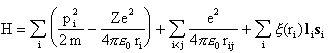The corresponding Hamilton operator is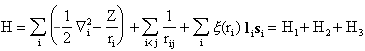H1 is uncomplicated, leading to a system of uncoupled differential equations which may be solved using a wavefunction that is a product of one-electron wavefunctions in the usual manner. At the moment, we also restrict the discussion to atoms which are not too heavy, which allows us to treat H3 as a perturbation. Remaining is H2, which we know leads to coupled differential equations. However, H2 may be separated into two parts, one of which is centrally symmetric. In a first approximation, this part is maintained along with H1 in the Schrödinger equation. This is the important central field approximation, which may be stated as follows:

In the central field approximation, each electron moves independently of the other electrons in the atom in a spherically -symmetric average field created by the nucleus and the other electrons.

This approximation provides a much better description of the atom than if only H1 is considered. The Hamiltonian may for each electron be writtenwhere the potential energy operator V(ri) is spherically symmetric. The Hamiltonian reduces to a sum of one-electron operators, each giving rise to a one-electron eigenfunction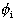, which is also referred to as an orbital. For each one-electron operator Hi an eigenvalue equation can be formulated asThe wavefunction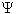for the Hamilton operator H0 may then be written in terms of a product of one-electron functions, i.e.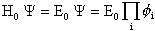while the energy eigenvalue E0 of H0 is obtained as a sum of one-electron energies, i.e.Since the potential is spherically symmetric, each wavefunctionwill as in the one-electron case be an eigenfunction to the operators li2, si2, liz and siz. The state for each electron is therefore defined by the four quantum numbers n, l, ml, ms or n, l, j, m dependent upon which representation is preferred.

The energy for each electron is determined solely by n and l, which means that the wavefunctions are degenerate with respect to ml and ms. According to the Pauli exclusion principle we may therefore have a maximum of 2(2l+1) electrons with the same n and l.

The 2(2l+1) electrons with the same n and l are said to form a closed shell, often called subshell. All closed shells form symmetric charge distributions.Last change: 19 February 2001